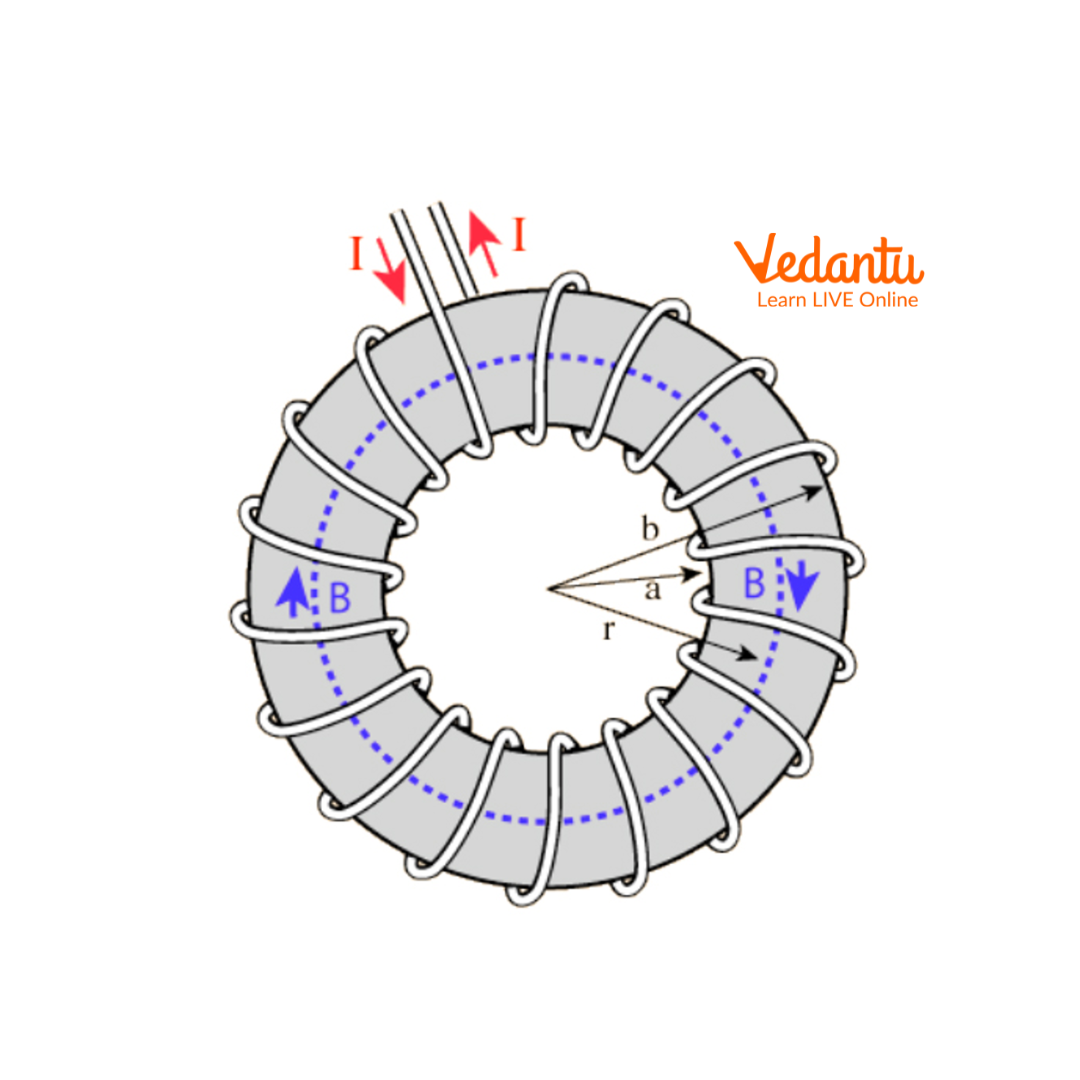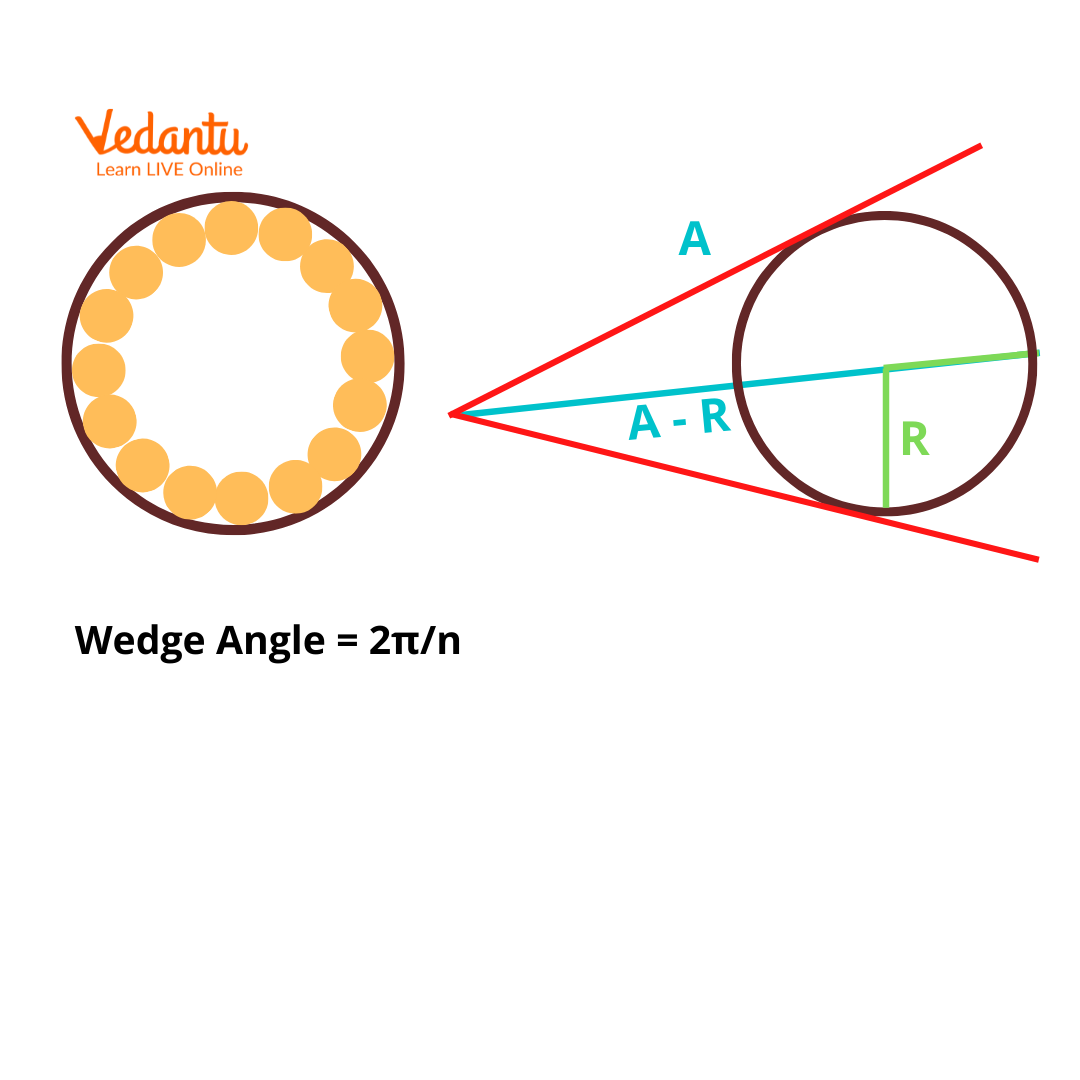Courses
Courses for Kids
Free study material
Free LIVE classes
More

# Magnetic Field Due To Toroid for JEE## What is a Toroid?

Last updated date: 23rd Mar 2023
Total views: 64.2k
Views today: 1.41k

A toroid is a hollow circular ring that looks like a doughnut. It has many numbers of turns of wires that are wound so close that there is a negligible amount of space between any two turns. They are similar to solenoids and are used in applications requiring high frequencies. In general, toroids act as inductors in electric circuits.

In this article, we will discuss about toroid, magnetic field due to toroid and comparison of a toroid and solenoid and answer questions such as “how can you increase the magnetic field inside a toroid ?” in detail.

## Ampere’s Circuital Law

According to Ampere’s Circuital Law, “the line integral of the magnetic field surrounding closed loops equals the number of times the algebraic sum of currents passing through the loop.”

$\oint {B}.{dl}={\mu_0}N~I$

Where I is the current and μo is the permeability of free space.

## How Do Toroids Behave as Inductors?

When current passes through a toroid, a magnetic field is produced around it. The strength of the magnetic field depends on the amount of current that passes through the toroid. The flux of the produced magnetic field will depend on the number of concentric circles that are perpendicular to the current direction. These flux lines tend to form closed loops around the coil. Changes in the current will lead to changes in the magnetic flux which in turn induces an electromotive force. This emf is in the opposite direction to the voltage across the coil.

## Generation of a Magnetic Field due to a Toroid

Ampere Circuit Law governs the generation of the magnetic field in a toroid.Toroid Diagram

In the above toroid diagram, let B be the magnetic field present at a point inside the toroid. This point is at a radius r from the centre of the toroid. According to the right-hand thumb rule for circular loops, the direction of the magnetic field at this point (inside the toroid) is clockwise. Now, if we consider other points at radii a and b from the centre of the toroid, the magnetic field B present inside the toroid will be tangential to the magnetic fields at each of the other points.

Due to symmetry, the magnitude of the magnetic field at all points on the circular ring will be the same and the field will be tangential. Hence,

$\oint {B}.{dl}=B\oint {dl}=2\pi rB$

Say the number of turns in the coil is N, then the net current that passes through the coil becomes NI, where I is the amount of current in the toroid.

According to Ampere’s Law,

$\oint {B}.{dl}={\mu_0}{NI}$

$2 \pi r B={\mu_0}{NI}$

$B=\dfrac {{\mu_0}{NI}}{2 \pi r}$

This is known as the toroid magnetic field formula.

It can be observed that the magnetic field is not uniform throughout the cross-section but is a variable.

## Formula for Number of Turns in a Toroid

Let’s have a look at the diagram below. It shows the cross-section of the wires wound on the toroid.Cross-section of Wires Wound on the Toroid

Let R be the radius of the wire, n be the number of loops and A be the inner radius of the toroid.

The wedge angle is 2π/n radians.

The equation that defines the relationship between R, A, and n is as follows:

$\sin \dfrac {\pi}{n}=\dfrac {R}{A-R} ~radians$

Using the above relation, we can find the number of turns in a toroid.

## Properties of a Toroid

• They exhibit high inductance due to the presence of a closed-loop.

• They have a very strong magnetic field.

• The emitted electromagnetic interference in toroids is very low.

• When compared to their equivalent solenoid coils, toroids have high inductance and high Q factors.

## Principle of a Toroidal Transformer

One of the most critical applications of the toroid is in toroidal transformers, commonly found in many household appliances. It is used to isolate and transform power. The working principle of a toroidal transformer is as follows:

1. An AC voltage is applied to the primary coil on both ends.

2. As a result of this AC voltage, an alternating current and a corresponding magnetic flux change is generated in the secondary coil of the toroidal transformer.

3. A mutual induction in the secondary coil and self-induction in the primary coil takes place.

4. The direction of the self-induced emf in the primary coil is opposite in direction to the applied AC voltage.

5. Certain power consumption is required to maintain the magnetic flux in the secondary coil. No-load current i.e., the current present in the primary coil when the secondary coil is not connected to any type of load exists at this point in time.

6. Once the secondary coil is connected to a load, a current will be generated leading to magnetic flux in the opposite direction.

7. After this point, the increase in the secondary coil current will lead to the maintenance of the total magnetic flux present in the iron core.

8. Electrical to magnetic energy conversion and vice versa happens in one of each winding of the toroidal transformer.

## Similarities Between Toroid and Solenoid

1. Both the solenoid and the toroid are designed based on electromagnetism.

2. When an electric current is applied, they both behave like electromagnetic materials.

3. The magnetic field inside the solenoid and the toroid are the same.

## Differences Between Toroid and Solenoid

 Property Solenoid Toroid Shape Cylindrical Circular Location of magnetic field formation Outside Inside Uniformity of magnetic field Uniform Non-uniform

## Summary

Toroids are hollow circular ring types tightly wound by a number of wires. Toroids are used as inductors in low-frequency cases. Few major applications of toroids include refrigerators, medical devices, telecommunications, industrial control, musical instruments, air conditioners, amplifiers, etc.

## FAQs on Magnetic Field Due To Toroid for JEE

1. Which is more efficient, a solenoid or a toroid? Explain.

Toroids are generally highly efficient since the loss due to flux leakage is minimal. This is because of the fact that the wires in a toroid are wound so tightly that there exists negligible space between any two wires. Also, their cylindrical shape contributes to their efficiency. Being a closed-loop inductor, a toroid has better or higher efficiency than open-loop type inductors like solenoids.

2. What is the difference in magnetic field between a solenoid and a toroid?

The shapes of both the coils mentioned are different. A solenoid appears to be wrapped around a cylinder while a toroid is circular. The magnetic field forms outside the solenoid and inside its value is zero. The opposite happens for the toroid where the magnetic field is inside. Also, the toiroid creates a non-uniform magnetic field while the solenoid creates a uniform field. The strength of the magnetic field due to a toroid is stronger than the magnetic field due to a solenoid.